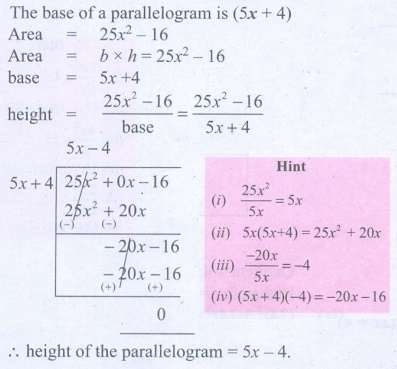Home | | Maths 9th std | Exercise 3.7: Division of Polynomials

# Exercise 3.7: Division of Polynomials

Maths : Algebra: Book Back, Exercise, Example Numerical Question with Answers, Solution : Exercise 3.7: Division of Polynomials

Exercise 3.7: Division of Polynomials

1. Find the quotient and remainder of the following.

(i) (4x3 + 6x2 – 23x +18) ÷ (x+3)(ii) (8y3 – 16y2 + 16y –15) ÷ (2y–1)(iii) (8x3 – 1) ÷ (2x–1)(iv) (–18z + 14z2 + 24z3 +18) ÷ (3z+4)2. The area of a rectangle is x2 + 7x + 12. If its breadth is (x+3), then find its length.3. The base of a parallelogram is (5x+4). Find its height, if the area is 25x2–16.4. The sum of (x+5) observations is (x3+125). Find the mean of the observations.5. Find the quotient and remainder for the following using synthetic division:

(i) (x3 + x2 7x 3) ÷(x 3) (ii) (x 3 + 2x2 x 4) ÷(x + 2)

(iii) (3x 3 2x2 + 7x 5) ÷(x + 3) (iv) (8x 4 2x2 + 6x + 5) ÷(4x +1)6. If the quotient obtained on dividing (8x 4 2x2 + 6x 7) by (2x +1) is (4x 3 + px 2 qx + 3) , then find p, q and also the remainder.7. If the quotient obtained on dividing 3x3 + 11x2 + 34x +106 by x − 3 is 3x2 + ax +b , then find a, b and also the remainder.Tags : Numerical Problems with Answers, Solution | Algebra | Maths , 9th Maths : UNIT 3 : Algebra
Study Material, Lecturing Notes, Assignment, Reference, Wiki description explanation, brief detail
9th Maths : UNIT 3 : Algebra : Exercise 3.7: Division of Polynomials | Numerical Problems with Answers, Solution | Algebra | Maths